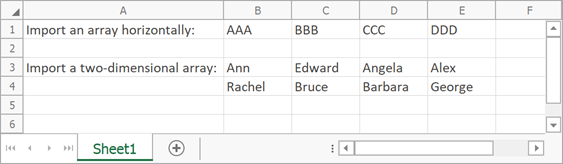The requested page is not available for the requested platform. You are viewing the content for .NET Framework 4.5.2+ platform.

## Mobile

19.2
19.1
18.2
18.1
17.2
.NET Framework 4.5.2+
.NET Framework 4.5.2+
.NET Standard 2.0+
.NET Core 3.0+

# WorksheetExtensions.Import(Worksheet, Object[], Int32, Int32, Boolean) Method

Imports data from an one-dimensional array of objects.

You require a license to the DevExpress Office File API or DevExpress Universal Subscription to use this method in production code.

Assembly: DevExpress.Docs.v19.2.dll

## Declaration

``````public static void Import(
this Worksheet sheet,
object[] array,
int firstRowIndex,
int firstColumnIndex,
bool isVertical
)``````
``````<ExtensionAttribute>
Public Shared Sub Import(
sheet As Worksheet,
array As Object(),
firstRowIndex As Integer,
firstColumnIndex As Integer,
isVertical As Boolean
)``````
``````public static void Import(
this Worksheet sheet,
object[] array,
int firstRowIndex,
int firstColumnIndex,
bool isVertical
)``````
``````<ExtensionAttribute>
Public Shared Sub Import(
sheet As Worksheet,
array As Object(),
firstRowIndex As Integer,
firstColumnIndex As Integer,
isVertical As Boolean
)``````
``````public static void Import(
this Worksheet sheet,
object[] array,
int firstRowIndex,
int firstColumnIndex,
bool isVertical
)``````
``````<ExtensionAttribute>
Public Shared Sub Import(
sheet As Worksheet,
array As Object(),
firstRowIndex As Integer,
firstColumnIndex As Integer,
isVertical As Boolean
)``````

## Parameters

Name Type Description
sheet Worksheet

A Worksheet that is the worksheet to which the data is imported.

array Object[]

A one-dimensional array of objects that is the data source.

firstRowIndex Int32

An integer that is the row index of the start cell in which the imported data will be inserted.

firstColumnIndex Int32

An integer that is the column index of the start cell in which the imported data will be inserted.

isVertical Boolean

true to insert imported data vertically; otherwise, false

## Parameters

Name Type Description
sheet Worksheet

A Worksheet that is the worksheet to which the data is imported.

array Object[]

A one-dimensional array of objects that is the data source.

firstRowIndex Int32

An integer that is the row index of the start cell in which the imported data will be inserted.

firstColumnIndex Int32

An integer that is the column index of the start cell in which the imported data will be inserted.

isVertical Boolean

true to insert imported data vertically; otherwise, false

## Parameters

Name Type Description
sheet Worksheet

A Worksheet that is the worksheet to which the data is imported.

array Object[]

A one-dimensional array of objects that is the data source.

firstRowIndex Int32

An integer that is the row index of the start cell in which the imported data will be inserted.

firstColumnIndex Int32

An integer that is the column index of the start cell in which the imported data will be inserted.

isVertical Boolean

true to insert imported data vertically; otherwise, false

## Remarks

The Import imports data from a one-dimensional array and enables you to specify how to place array data in cells - vertically or horizontally.

The following code snippet illustrates how to use the Import method.

``````' Create an array containing string values.
Dim array() As String = { "AAA", "BBB", "CCC", "DDD" }

' Import the array into the worksheet and insert it horizontally, starting with the B1 cell.
worksheet.Import(array, 0, 1, False)

' Create a two-dimensional array containing string values.
Dim names(,) As String = {
{"Ann", "Edward", "Angela", "Alex"},
{"Rachel", "Bruce", "Barbara", "George"}
}

' Import the two-dimensional array into the worksheet and insert it, starting with the B3 cell.
worksheet.Import(names, 2, 1)
``````

The image below shows the results.